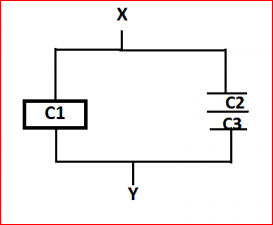# Capacitor with dielectric

• gracy

## Homework Statement

Calculate the capacitance of the parallel plate capacitor shown in the following figure,between points X and Y.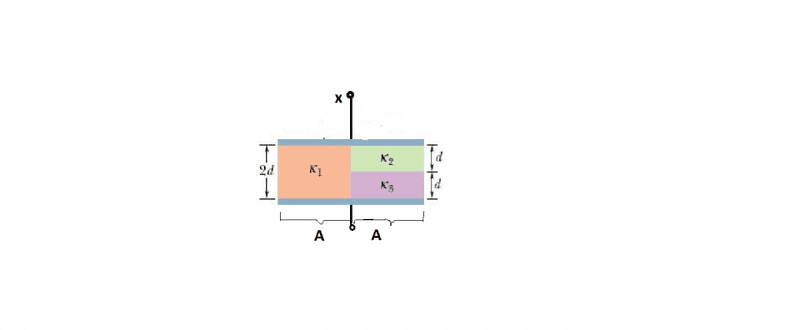## Homework Equations

##C##=##\frac{Kε0A}{d}##

## The Attempt at a Solution

Which capacitor has an area "A"?
I will have to transform this into circuit diagram.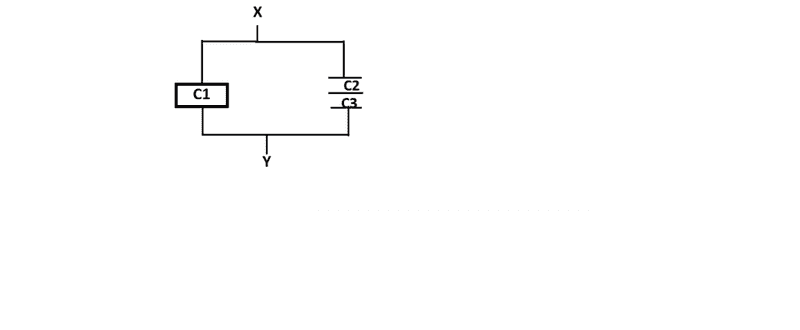Yes, calculate using your 'transformed' equivalent. I would not assume the dimension A to be of an area unless that were clearly stated somewhere.

I would not assume the dimension A to be of an area unless that were clearly stated somewhere.
Yes it is mentioned that A=area

Please show the original figure and text, not the one, modified by you.

Please show the original figure and text, not the one, modified by you.
Calculate the capacitance of the parallel plate capacitor shown in the following figure,between points X and Y.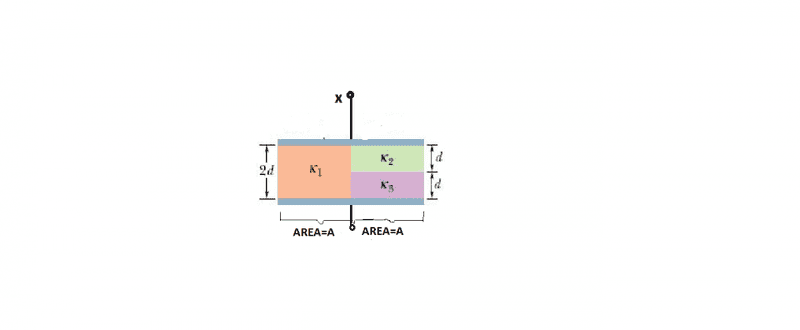Show the real figure in the book, without modifying it.

Show the real figure in the book, without modifying it.
That's what it is.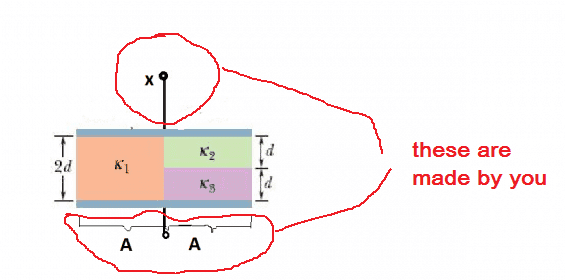You show what areas are A, then ask "Which capacitor has an area "A"?" in the OP. Where is A in the original picture? Where are X and Y in the original picture?

Don't see attached file.

Sorry
Now this one should be perfect.This is what I have in my textbook.
View attachment 93303
I think the transformed circuit diagram by you is correct. Both the capacitors are having area A. You'll have to calculate the equivalent capacitance of the composite capacitor (the one with dielectric constants k2 and k3).

Both the capacitors are having area A.
But there are 3 capacitors ,right?

But there are 3 capacitors ,right?
I'll prefer to call the capacitor on the right as a single capacitor with veriable dielectric constant. There is no metal connection between the dielectrics k2 and k3. So, I don't think they can be a series combination.

Last edited:
Although there is no metal plate shown separating the different dielectrics, nothing would effectively change were you to include a third parallel metal plate as a separator because it would all be along a line of equipotential. So you can indeed analyse it as a series pair of capacitors.

•cnh1995
You're right. Seeing the answer I've got using the composite dielectric method, it turns out that it is effectively a series combination of the two.

But what about area?The question does not clearly mention which capacitor has an area A?From the diagram,the total area is 2A. So, when you split this capacitor into two( or three) capacitors, the area of each capacitor will be A.

From the diagram,the total area is 2A. So, when you split this capacitor into two( or three) capacitors, the area of each capacitor will be A.
How in case of three capacitors the area of each capacitor will be A?

How in case of three capacitors the area of each capacitor will be A?
I believe it means that the area of each side of every metal plate of the equivalent three capacitors here is A.

But what about area?The question does not clearly mention which capacitor has an area A?
In the case of your equivalent set of three capacitors, each capacitor, C1, C2, and C3 has area A.